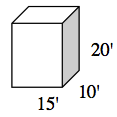### Home > CCG > Chapter 9 > Lesson 9.2.1 > Problem9-69

9-69.

A butterfly house at a local zoo is a rectangular prism with dimensions $20' × 15' × 10'$ and contains $625$ butterflies.

1. Sketch the prism on your paper.2. What is the volume of the butterfly house? Show your work.

3. How many cubic feet of air is there for each butterfly?

Use the volume from part (b), and the total number of butterflies to find how much space belongs to each butterfly
if the space in the house is divided evenly.

4. Density is the quantity of something per unit measure, especially length, area, or volume. For example you might talk about the density of birds on a power wire (maybe a flock lands with $7$ birds/meter), population density (the density of Singapore is $7301$ people per square kilometer), or the mass density of an element (iron has density of $7.874\frac{\text{g}}{\text{cm}^3}$).

Assuming the butterflies are equally distributed inside the butterfly house, what is the density of butterflies? Explain.